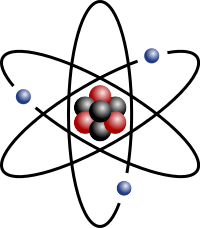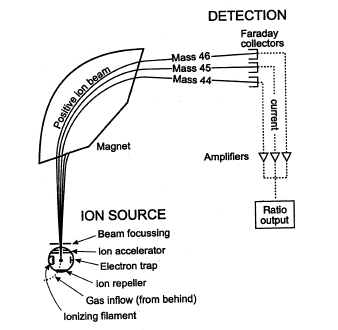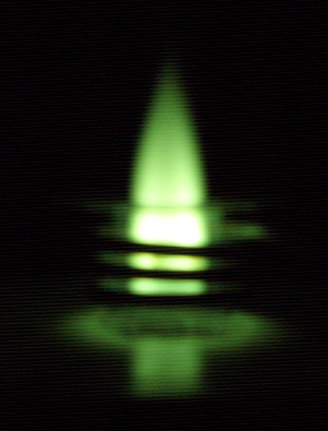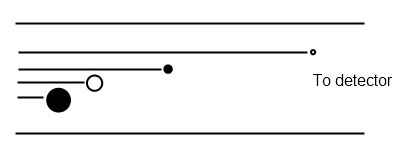## Average Atomic Mass

The average atomic mass of an element is the sum of the masses of its isotopes, each multiplied by its natural abundance.

### Learning Objectives

Calculate the average atomic mass of an element given its isotopes and their natural abundance

### Key Takeaways

#### Key Points

• An element can have differing numbers of neutrons in its nucleus, but it always has the same number of protons. The versions of an element with different neutrons have different masses and are called isotopes.
• The average atomic mass for an element is calculated by summing the masses of the element’s isotopes, each multiplied by its natural abundance on Earth.
• When doing any mass calculations involving elements or compounds, always use average atomic mass, which can be found on the periodic table.

#### Key Terms

• mass number: The total number of protons and neutrons in an atomic nucleus.
• natural abundance: The abundance of a particular isotope naturally found on the planet.
• average atomic mass: The mass calculated by summing the masses of an element’s isotopes, each multiplied by its natural abundance on Earth.

The atomic number of an element defines the element’s identity and signifies the number of protons in the nucleus of one atom. For example, the element hydrogen (the lightest element) will always have one proton in its nucleus. The element helium will always have two protons in its nucleus.

### Isotopes

Atoms of the same element can, however, have differing numbers of neutrons in their nucleus. For example, stable helium atoms exist that contain either one or two neutrons, but both atoms have two protons. These different types of helium atoms have different masses (3 or 4 atomic mass units ), and they are called isotopes. For any given isotope, the sum of the numbers of protons and neutrons in the nucleus is called the mass number. This is because each proton and each neutron weigh one atomic mass unit (amu). By adding together the number of protons and neutrons and multiplying by 1 amu, you can calculate the mass of the atom. All elements exist as a collection of isotopes. The word ‘isotope’ comes from the Greek ‘isos’ (meaning ‘same’) and ‘topes’ (meaning ‘place’) because the elements can occupy the same place on the periodic table while being different in subatomic construction.Lithium Atom: Stylized lithium-7 atom: 3 protons (red), 4 neutrons (black), and 3 electrons (blue). (Lithium also has another, rarer isotope with only 2 neutrons.)

### Calculating Average Atomic Mass

The average atomic mass of an element is the sum of the masses of its isotopes, each multiplied by its natural abundance (the decimal associated with percent of atoms of that element that are of a given isotope).

Average atomic mass = f1M1 + f2M2 +… + fnMn where f is the fraction representing the natural abundance of the isotope and M is the mass number (weight) of the isotope.

The average atomic mass of an element can be found on the periodic table, typically under the elemental symbol. When data are available regarding the natural abundance of various isotopes of an element, it is simple to calculate the average atomic mass.

• For helium, there is approximately one isotope of Helium-3 for every million isotopes of Helium-4; therefore, the average atomic mass is very close to 4 amu (4.002602 amu).
• Chlorine consists of two major isotopes, one with 18 neutrons (75.77 percent of natural chlorine atoms) and one with 20 neutrons (24.23 percent of natural chlorine atoms). The atomic number of chlorine is 17 (it has 17 protons in its nucleus).

To calculate the average mass, first convert the percentages into fractions (divide them by 100). Then, calculate the mass numbers. The chlorine isotope with 18 neutrons has an abundance of 0.7577 and a mass number of 35 amu. To calculate the average atomic mass, multiply the fraction by the mass number for each isotope, then add them together.

Average atomic mass of chlorine = (0.7577 $\cdot$ 35 amu) + (0.2423 $\cdot$ 37 amu) = 35.48 amu

Another example is to calculate the atomic mass of boron (B), which has two isotopes: B-10 with 19.9% natural abundance, and B-11 with 80.1% abundance. Therefore,

Average atomic mass of boron = (0.199 $\cdot$ 10 amu) + (0.801 $\cdot$  11 amu) = 10.80 amu

Whenever we do mass calculations involving elements or compounds (combinations of elements), we always use average atomic masses.

## Mass Spectrometry to Measure Mass

Mass spectrometry is a powerful characterization method that identifies elements, isotopes, and compounds based on mass-to-charge ratios.

### Learning Objectives

Define the primary application of a mass spectrometer

### Key Takeaways

#### Key Points

• Mass spectrometers work on samples in a gaseous state.
• The gaseous samples are ionized by an ion source, which adds or removes charged particles ( electrons or ions). Examples of ion sources include inductively coupled plasma and electron impact.
• Mass analyzers separate ionized samples according to their mass-to-charge ratio. Time-of-flight and quadrupole are examples of mass analyzers.
• A particle’s mass can be calculated very accurately based on parameters such as how long it takes to travel a certain distance or its angle of travel.
• Mass spectrometers are so accurate that they can determine the types of elements in a compounds or measure the differences between the mass of different isotopes of the same atom.

#### Key Terms

• ionization: Any process that leads to the dissociation of a neutral atom or molecule into charged particles (ions).
• plasma: A state of matter consisting of partially ionized gas, usually at high temperatures.
• mass-to-charge ratio: The best way to separate ions in a mass spectrometer. This number is calculated by dividing the ions weight by its charge.

Mass spectrometry (MS) is a powerful technique that can identify a wide variety of chemical compounds. It is used to determine a particle’s mass, the elemental composition of a sample, and the chemical structures of larger molecules.

Mass spectrometers separate compounds based on a property known as the mass-to-charge ratio: the mass of the atom divided by its charge. First, the sample is ionized. Ionization is the process of converting an atom or molecule into an ion by adding or removing charged particles such as electrons or ions. Once the sample is ionized, it is passed through some form of electric or magnetic field. A particle’s mass can be calculated based on parameters such as how long it takes to travel a certain distance or its angle of travel.Schematic of Mass Spectrometer: A sample is loaded onto the mass spectrometer, where it undergoes vaporization and ionization. The components of the sample are ionized by one of a variety of methods, such as the ionizing filament. The ions are separated in an analyze by magnetic fields. They are separated according to their mass-to-charge ratios. The ions are detected, usually by a quantitative method such as a Faraday collector. The ion signal is processed into a mass spectrum.

### The Make-Up of Mass Spectrometry (MS) Instruments

MS instruments consist of two main components:

• An ion source, which can convert sample molecules into ions
• A mass analyzer, which sorts the ions by mass by applying electromagnetic fields

There are a wide variety of techniques for ionizing and detecting compounds.

### Ionizing CompoundsInductively coupled plasma (ICP) flame: Picture of an ICP flame viewed through green welder’s glass.

The ion source is the part of the mass spectrometer that ionizes the compound. Depending on the information desired from mass spectrometry analysis, different ionization techniques may be used. For example, the most common ion source for analyzing elements is inductively coupled plasma (ICP). In ICP, a 10,000-degree C “flame” of plasma gas is used to atomize sample molecules and strip the outer electrons from those atoms.

The plasma is usually generated from argon gas. Plasma gas is electrically neutral overall, but a substantial number of its atoms are ionized by the high temperature.

Electron impact (EI) is another method for generating ions. In EI, the sample is heated until it becomes a gas. It is then passed through a beam of electrons. This high-energy beam strips electrons from the sample molecules, leaving behind a positively charged radical species.

### Mass Analyzers

Mass analyzers separate the ions according to their mass-to-charge ratios. There are many types of mass analyzers. Each has its strengths and weaknesses, including:

• how accurately they can measure similar mass-to-charge ratios
• the range of masses and sample concentrations they can measure.

For example, a time-of-flight (TOF) analyzer uses an electric field to accelerate the ions through the same potential and then measures the time they take to reach the detector. Since the particles all have the same charge, their velocities depend only on their masses, and lighter ions will reach the detector first.Time-of-Flight mass analyzer: Schematic of a time-of-flight (TOF) mass analyzer.

Another type of detector is a quadrupole. Here, ions are passed through four parallel rods, which apply a varying electric voltage. As the field changes, ions respond by following complex paths. Depending on the applied voltage, only ions of a certain mass-to-charge ratio will pass through the analyzer. All other ions will be lost by collision with the rods.

### Using a Mass Spectrometer to Measure Mass

Here is how a mass spectrometer would analyze a sample of sodium chloride (table salt).

• In the ion source, the sample is vaporized (turned into gas) and ionized into sodium (Na+) and chloride (Cl) ions.
• Sodium atoms and ions have only one isotope and a mass of about 23 amu.
• Chloride atoms and ions come in two isotopes, with masses of approximately 35 amu (at a natural abundance of about 75 percent) and approximately 37 amu (at a natural abundance of about 25 percent).
• The mass analyzer part of the spectrometer contains electric and magnetic fields, which exert forces on ions traveling through these fields. The angle at which the ion moves through the fields depends on its mass-to-charge ratio: lighter ions change direction more than heavier ions.
• The streams of sorted ions pass from the analyzer to the detector, which records the relative abundance of each ion type. This information is used to determine the chemical composition of the original sample (i.e. that both sodium and chlorine are present in the sample) as well as its isotopic composition (the ratio of chlorine-35 to chlorine-37).